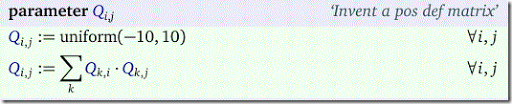## Monday, January 30, 2017

### Little trick: random positive definite matrix

Sometimes we want to test a model with some random data. When dealing with quadratic problems we may need to invent a positive definite matrix $$Q$$. One simple way to do this is as follows:I.e. in matrix terms: $$Q:=Q^TQ$$ (i.e. the assignment above is not done one-by-one but rather in parallel). To be precise: $$Q$$ will be positive semi-definite, i.e. $$x^TQx\ge 0$$.

This simple device can help generating random data for convex quadratic models.